Practice questions available in McGraw Hill Math Grade 6 Answer Key PDF Lesson 11.3 Adding and Subtracting Money will engage students and is a great way of informal assessment.

Question 1.Answer: $10.67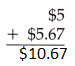So, the sum of two numbers$5 and $5.67 is$10.67

Question 2.Answer: $137.01So, the sum of two numbers 3.45 and 133.56 is$137.01

Question 3.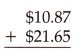Answer: $32.52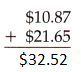So, the sum of two numbers$10.87 and $21.65 is$32.52

Question 4.Answer: $6.45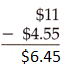The difference of$11 and $4.55 is$6.45

Question 5.Answer: $2.89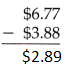The difference of 6.77 and 3.88 is$2.89

Question 6.Answer: $6.85The difference of$12.30 and $5.45 is$6.85

Question 7.Answer: $5.11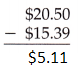The difference of 20.50 and 15.39 is 5.11 Question 8.Answer:$54.78The difference of 62.32 and 7.54 is 54.78

Question 9.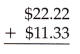Answer: $33.55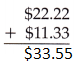The sum of 22.22 and 11.33 is$33.55

Question 10.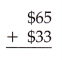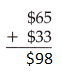By adding 65 and 33 we get 98.

Question 11.Answer: $8.62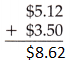By adding 5.12 and 3.50 we get$8.62

Question 12.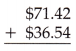Answer: $107.96By adding 71.42 and 36.54 we get$107.96

Question 13.Answer: $687.20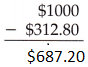The difference of 1000 and 312.80 is 687.2 Question 14.Answer:$9.77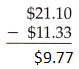The difference of 21.10 and 11.33 is $9.77 Question 15.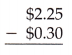Answer:$1.95The difference of 2.25 and 0.30 is $1.95 Question 16.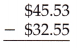Answer:$12.98The difference of 45.53 and 32.55 is $12.98 Question 17.Answer:$0.32The difference of $12.30 and$11.98 is $0.32 Question 18.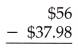Answer:$18.02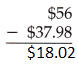The difference of 56 and 37.98 is $18.02 Question 19.Answer:$21The difference of 33 and 12 is $21. Question 20.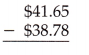Answer:$2.87The difference of 41.65 and 38.78 is \$2.87

Scroll to Top